# CalculusIn this topic we will look at

• Rates of Change
• Tangents and Normals
• Chain Rule
• Product and Quotient Rule
• Local Maximum and Minimum Points and Points of Inflexion
• Applications and Optimisation
• Indefinite Integration
• Definite Integration
• Areas between Graphs and Volumes
• Kinematics
• Integration by Substitution
• Integration by Parts

•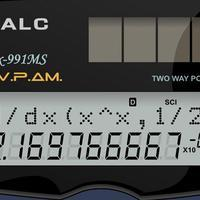#### SL Mixed Differentiation

This page is ideal for practising all the skills of differentiation. There are a series of quizzes that you can work through. You may wish to use this page in preparation in preparation for a test on this topic or the final or practice examinations.

•#### HL Mixed Differentiation

This page is ideal for practising all the skills of differentiation. You may wish to use this page in preparation for a test on this topic or for the final examinations. The quizzes on this page have been carefully created to take you through...

•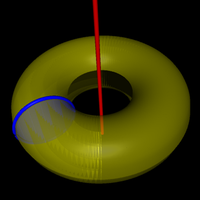#### Mixed Integration

This page is ideal for practising all the skills of integration. You may wish to use this page in preparation for a test on this topic or for the final examinations. The quizzes on this page have been carefully created to take you through all the skills th

•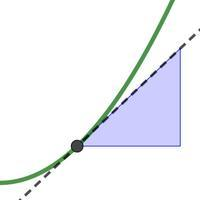#### Introducing Derivatives

Differentiation allows to find rates of change. The derivative is the rate at which one quantity changes with respect to an other. When we differentiate a function we find the gradient function. On a graph, the derivative is the slope of...

•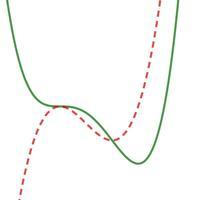#### Graphs and Derivatives

On the following page, we will look at graphs and derivatives. We will get some practice in sketching gradient functions and we will carefully consider stationary points (maximum, minimum and points of inflexion) as well as non-stationary points of inflexi

•#### Chain Rule

The Chain Rule is used for differentiating composite functions. The rule itself looks really quite simple (and it is not too difficult to use). The most important thing to understand is when to use it and then get lots of practice. It is...

•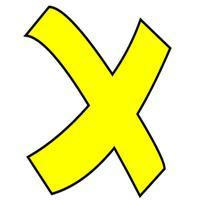#### Product and Quotient Rule

The Product Rule is a formula that we can use to differentiate the product of 2 (or more) functions. The Quotient Rule is for the quotient of two functions (one function divided by another). The rules are quite easy to apply. The challenge...

•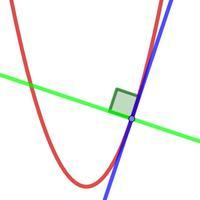#### Equation of Tangent and Normal

On this page we look at how to find the equation of a tangent and also the normal to a curve. A tangent is a straight line that touches a curve at one point and has the same gradient as the curve at that point. A normal is straight line...

•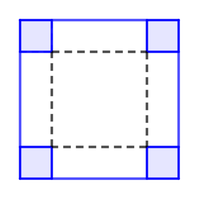#### Optimisation

Optimisation questions involve finding the best solution to a problem. Usually, that is the maximum or minimum value of a function. Questions on this topic require you to take information from a practical problem and write this as a function, then using di

•#### Implicit Differentiation

This page is all about implicit equations and how we find the gradient of them. Implicit equations are equations which are not written explicitly – I’ll explain later! Examination questions typically ask you to find the equation of a...

•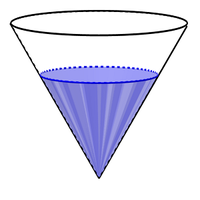#### Related Rates of Change

This page is about finding related rates of change, otherwise know as connected rates of change. In questions on this topic, you will be given a rate of change of some quantity and be required to find the rate of change of another, that is...

•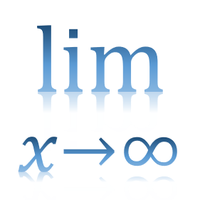#### L'Hôpital's Rule

You will probably have already considered limits in work on the sum of a geometric series, rational functions and asymptotes and differentiation from first principles. In this page, we will take the idea of limits further. L'Hôpital's rule...

•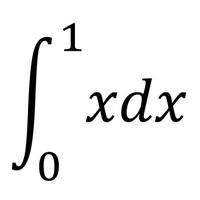#### Definite Integration

Integration is sometimes called antidifferentiation, as it is the opposite process of differentiation. When we find an indefinite integral we find a function with an abritrary constant, C. When we find a definite integral, we find a numerical value.

•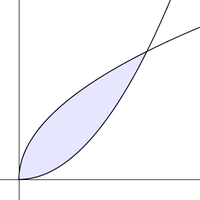#### Area between Graphs SL

On this page, we'll look at how we can use integration to find areas. This is a really common question in examinations.Â  The technique is not too hard, but there are just a couple of pitfalls to avoid which will be explained. There are 3...

•#### Area between Graphs HL

On this page, we'll look at how we can use integration to find areas. This is a really common question in examinations.  The technique is not too hard, but there are just a couple of pitfalls to avoid which will be explained. There are...

•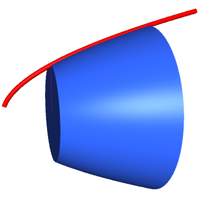#### Volume of Revolution

In this page, we will learn about how to find the volume generated by rotating a region around the x axis and the y axis. The formule for these are not difficult to use. The difficulty often comes with applying the integration techniques. It...

•#### Integration by Substitution SL

Integration by substitution or U-substitution is a method that will help you integrate many different functions. By changing the variable of the integrand, we can make an apparently difficult problem into a much simpler one. The challenge is...

•#### Integration by Substitution HL

Integration by substitution or U-substitution is a method that will help you integrate many different functions. By changing the variable of the integrand, we can make an apparently difficult problem into a much simpler one. The challenge is recognising wh

•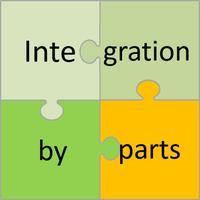#### Integration by Parts

Integration by parts is a method of integration that we use to integrate the product (usually !) of two functions. The aim is to change this product into another one that is easier to integrate. Although the formula looks quite odd at first glance, the tec

•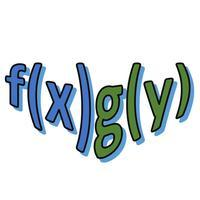#### Differential Equations - Separable Variables

If you want to describe the world around you, be it the forces acting on a body, the growth of a virus or the temperature of the coffee in your cup, you will be dealing with differential equations. On this page we will look at the simplest type: differenti

•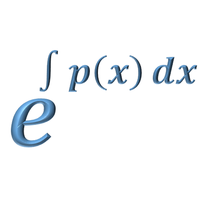#### Differential Equations - Integrating Factor

On this page we will look at another type of differential equations: linear differential equations in the form y' + P(x)y=Q(x) which can be solved by using an integrating factor. There are many applications of this type of differential equation....

•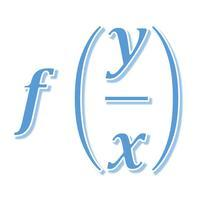#### Differential Equations - Homogeneous

When a first order differential equation is not separable, nor linear (integrating factor), it may still be possible to solve it analytically using a substitution. This will work when the equation is homogeneous. A homogenous differential equation...